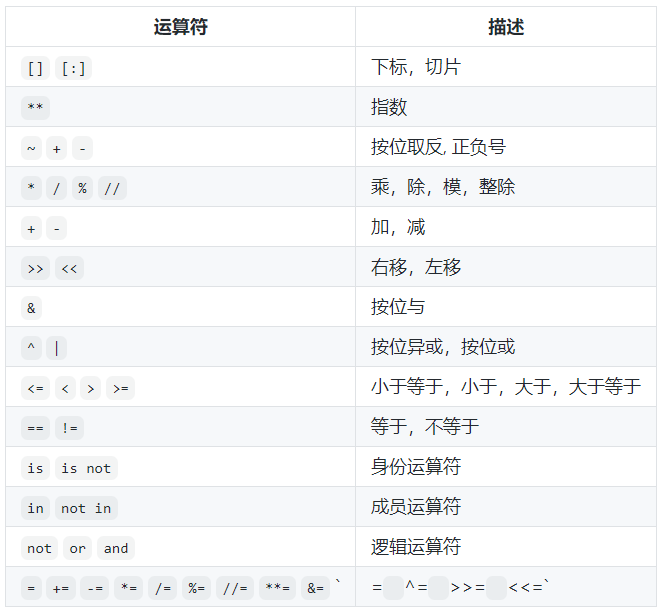# Day02 – 语言元素

### 一、变量

1. 变量的类型

• 整型（int）：在Python2.x中有`int``long`两种类型，但区别意义不大，所以在Python3.x中只保留了`int`一种。

• 浮点型（float）：在Python中，除了支持数学写法，还支持科学计数法。

• 字符串型（str）：以单引号或双引号括起来的任意文本。

• 布尔型（bool）：布尔值只有`True``False`两种值，即真和假。

• 复数型（complex）：即a+bj，注意复数用j表示

2. 变量命名规则

变量命名规则整体上与C语言没什么差别，在Python可以用中文作为函数名以及变量名，不过在编写程序过程中，应当有个好的命名习惯。

• 硬性规则：

• 变量名由字母（广义的Unicode字符，不包括特殊字符）、数字和下划线构成，数字不能开头。

• 大小写敏感（大写的a和小写的A是两个不同的变量）。

• 不要跟关键字（有特殊含义的单词，后面会讲到）和系统保留字（如函数、模块等的名字）冲突。

• 好的习惯：

• 用小写字母拼写，多个单词用下划线连接。

• 受保护的实例属性用单个下划线开头。

• 私有的实例属性用两个下划线开头。

3. 变量的使用

变量可以直接通过赋值的方式自动决定其类型，和MATLAB的方式差不多

``````a=123
b=4

print(a+b) # 127
print(a-b) # 119
print(a*b) # 492
print(a/b) # 30.75``````

Python中可以使用`type`函数来返回变量的类型，便于检查。

``````a=12
b=3.123
c='hello'

print(type(a)) #
print(type(b)) #
print(type(c)) # ``````

变量的类型转换

• `int()`:转换为整数,可指定进制
• `float()`:转换为浮点数
• `str()`:转换为字符串,可指定编码
• `chr()`:将整数转换为对应字符(ASCII)
• `ord()`:将字符转换为对应编码(整数)

示例：

``````a = int(input('a='))
b = int(input('b='))
print('%d + %d = %d' % (a,b,a+b))
print('%d - %d = %d' % (a,b,a-b))
print('%d * %d = %d' % (a,b,a*b))
print('%d / %d = %f' % (a,b,a/b))
print('%d // %d = %d' % (a,b,a//b)) # //表示整数除法
print('%d %% %d = %d' % (a,b,a%b)) # 在引号中%%表示一个%，区分%d
print('%d ** %d = %d' % (a,b,a**b)) # **表示几次方``````

运行后发现，好像Python的print倒不如c语言的printf好用，调用整型变量时一样是使用%d，但是注意后面需要用`%(参数，参数)`的形式

### 二、运算符

1. 常见运算符2. 赋值运算符`=` `+=` `*=`

3. 比较运算符`==` `!=` `<` `>` `<=` `>=`

4. 逻辑运算符`and` `or` `not`

对应C语言中的`&&``||``!`

### 三、练习

1. 华氏温度转换为摄氏温度

提示：华氏度到摄氏度的转换公式为：\$C=(F-32)/1.8\$

解：

``````f=float(input('请输入华摄度:'))
print('转换摄氏度的结果为: %.1f' % ((f-32)/1.8))``````
2. 输入圆的半径计算计算周长和面积

解：

``````pi=3.1416
r=float(input('输入半径:'))
print('半径为%.2f的圆的周长为%.2f，面积为%.2f' % (r,2*pi*r,pi*r**2))``````

Python中没有像C语言一样自带pi常量。。、

3. 输入年份判断是不是闰年

解：

使用布尔值作答

``````year=int(input('输入年份：'))
is_leap = year%4==0 and year%100!=0 or year%400==0
print(is_leap)``````

使用判断（可能目前超纲了哈哈）

``````year=int(input('输入年份：'))
if year%4==0 and year%100!=0 or year%400==0:
print('该年为闰年')
else:
print('不是闰年')``````